Important Questions: Coordinate Geometry

# Important Questions: Coordinate Geometry

Test Description

## 10 Questions MCQ Test Online MCQ Tests for Class 10 | Important Questions: Coordinate Geometry

Important Questions: Coordinate Geometry for Class 10 2022 is part of Online MCQ Tests for Class 10 preparation. The Important Questions: Coordinate Geometry questions and answers have been prepared according to the Class 10 exam syllabus.The Important Questions: Coordinate Geometry MCQs are made for Class 10 2022 Exam. Find important definitions, questions, notes, meanings, examples, exercises, MCQs and online tests for Important Questions: Coordinate Geometry below.
Solutions of Important Questions: Coordinate Geometry questions in English are available as part of our Online MCQ Tests for Class 10 for Class 10 & Important Questions: Coordinate Geometry solutions in Hindi for Online MCQ Tests for Class 10 course. Download more important topics, notes, lectures and mock test series for Class 10 Exam by signing up for free. Attempt Important Questions: Coordinate Geometry | 10 questions in 10 minutes | Mock test for Class 10 preparation | Free important questions MCQ to study Online MCQ Tests for Class 10 for Class 10 Exam | Download free PDF with solutions
 1 Crore+ students have signed up on EduRev. Have you?
Important Questions: Coordinate Geometry - Question 1

### If the distance between the points (2, - 2) and (-1, x) is 5, one of the values of x is

Detailed Solution for Important Questions: Coordinate Geometry - Question 1

(5)²= [{2-(-1)}² + {x-(-2)}²]
25= 9+(x+2)²
25-9 = (x+2)²
√16=x+2
x+2= 4
x=4-2
x= 2.

Important Questions: Coordinate Geometry - Question 2

### A triangle with vertices (4, 0), (- 1, - 1) and (3, 5) is a/an

Detailed Solution for Important Questions: Coordinate Geometry - Question 2

Let A(4, 0), B(-1, -1), C(3, 5)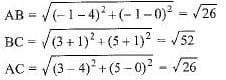⇒ AB2 + AC2 = BC2 and AB = AC
Hence, triangle is an isosceles right-angled triangle.

Important Questions: Coordinate Geometry - Question 3

### The points A (9, 0), B (9, 6), C (-9, 6) and D (-9, 0) are the vertices of a

Detailed Solution for Important Questions: Coordinate Geometry - Question 3

AB= √(9-9)²+(6-0)²=6
BC= √(-9-9)²+(6-6)²=18
CD = √(-9+9)²+(0-6)²=6
DA = √(-9-9)²+(0-0)²=18
so,it's opposite sides are equal,hence it is rectangle

Important Questions: Coordinate Geometry - Question 4

The points (- 4,0), (4, 0) and (0,3) are the vertices of a/an

Detailed Solution for Important Questions: Coordinate Geometry - Question 4

Isosceles triangle.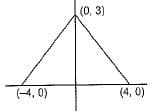Important Questions: Coordinate Geometry - Question 5

The distance between the points A (0, 6) and B (0, -2) is

Detailed Solution for Important Questions: Coordinate Geometry - Question 5

Since both these points lie on a straight line i.e x axis, distance will be the difference between the respective y coordinates
(0,-2) & (0,6)  =>  6-(-2)) = 6+2 = 8

Important Questions: Coordinate Geometry - Question 6

A circle drawn with origin as the centre passes through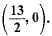The point which does not lie in the interior of the circle is

Detailed Solution for Important Questions: Coordinate Geometry - Question 6

Distance of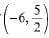from centre of the circle i. e.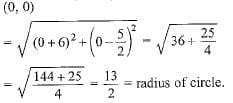Important Questions: Coordinate Geometry - Question 7

The distance between the points A (0, 7) and B (0, -3) is

Detailed Solution for Important Questions: Coordinate Geometry - Question 7

Since both these points lie on a straight line i.e x axis, distance will be the difference between the respective y coordinates

(0,-3)   (0,7)

7-(-3) = 7+3 = 10

Important Questions: Coordinate Geometry - Question 8

If the distance between the points (4,p) and (1,0) is 5 units, then the value of p is

Detailed Solution for Important Questions: Coordinate Geometry - Question 8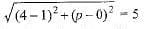⇒ 32 + p2 = 52 ⇒ p2 = 25 - 9 = 16 ⇒ p = ± 4

Important Questions: Coordinate Geometry - Question 9

The end points of diameter of circle are (2, 4) and (-3, -1). The radius of the circle is

Detailed Solution for Important Questions: Coordinate Geometry - Question 9

diameter = √((2-(-3))+ (4-(-1))2)

= √52+52 = √50 = 5√2

Important Questions: Coordinate Geometry - Question 10

If P(1, 2), Q(4,6), R(5,7) and S(a, b) are the vertices of a parallelogram PQRS, then

Detailed Solution for Important Questions: Coordinate Geometry - Question 10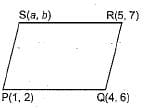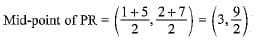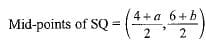Diagonals of parallelogram bisect each other.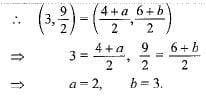## Online MCQ Tests for Class 10

456 tests
 Use Code STAYHOME200 and get INR 200 additional OFF Use Coupon Code
Information about Important Questions: Coordinate Geometry Page
In this test you can find the Exam questions for Important Questions: Coordinate Geometry solved & explained in the simplest way possible. Besides giving Questions and answers for Important Questions: Coordinate Geometry, EduRev gives you an ample number of Online tests for practice

456 tests